# Kaslo Bull Trout Productivity Analysis 2016

The suggested citation for this analytic report is:

Thorley, J.L. and Hogan P.M. (2017) Kaslo Bull Trout Productivity Analysis 2016. A Poisson Consulting Analysis Report. URL: http://www.poissonconsulting.ca/f/1827953676.

## Background

The Kaslo River and Keen Creek, which is a tributary of the Kaslo River, are important Bull Trout spawning and rearing tributaries on Kootenay Lake. From 2012 to 2016, field crews have night-snorkeled both systems in the fall and recorded all juvenile Bull Trout between 30 mm and 350 mm in length. Snorkel and electrofishing marking crews have also captured and tagged juvenile Bull Trout for the snorkel crews to resight. Redd counts have been conducted in both systems since 2006.

The primary goal of the current analyses is to answer the following questions:

What is the observer efficiency when night-snorkeling for juvenile Bull Trout in the Kaslo River and Keen Creek?

What are the densities of age-1 Bull Trout in the Kaslo River and Keen Creek?

What is the relationship between the number of redds and the resultant densities of age-1 Bull Trout two years later?

### Data Preparation

The data were provided in the form of clean and tidy Excel spreadsheets by Gary Pavan and prepared for analysis using R version 3.3.2 (R Core Team 2015).

Table 1. Bull Trout length cutoffs by age-class.

Age Class Length (mm)
Age-0 30 - 90
Age-1 91 - 150
Age-2+ 151 - 350

### Statistical Analysis

Model parameters were estimated using Maximum-Likelihood (ML) and Bayesian methods. The Maximum-Likelihood Estimates (MLE) were obtained using TMB (Kristensen et al. 2016) while the Bayesian estimates were produced using JAGS (Plummer 2015). For additional information on ML estimation the reader is referred to Millar (2011). For additional information on Bayesian modelling in the BUGS language, of which JAGS uses a dialect, the reader is referred to Kery and Schaub (2011).

Unless indicated otherwise, the Bayesian analyses used uninformative normal prior distributions (Kery and Schaub 2011, 36). The posterior distributions were estimated from 2,000 Markov Chain Monte Carlo (MCMC) samples thinned from the second halves of four chains (Kery and Schaub 2011, 38–40). Model convergence was confirmed by ensuring that $$\hat{R} < 1.1$$ (Kery and Schaub 2011, 40) for each of the monitored parameters (Kery and Schaub 2011, 61).

The parameters are summarised in terms of the point estimate, standard deviation (sd), the z-score, lower and upper 95% confidence/credible limits (CLs) and the p-value (Kery and Schaub 2011, 37, 42). For ML models, the point estimate is the MLE, the standard deviation is the standard error, the z-score is $$\mathrm{sd}/\mathrm{MLE}$$ and the 95% CLs are the $$\mathrm{MLE} \pm 1.96 \cdot \mathrm{sd}$$. A p-value of 0.05 indicates that the lower or upper 95% CL is 0. For Bayesian models, the estimate is the median (50th percentile) of the MCMC samples, the z-score is $$\mathrm{mean}/\mathrm{sd}$$ and the 95% CLs are the 2.5th and 97.5th percentiles.

The support for fixed terms in ML models with identical random (Kery and Schaub 2011, 75) effects was assessed using Akaike’s weights ($$w_i$$) calculated from the marginal Akaike’s Information Criterion corrected for small sample size (AICc, Burnham and Anderson 2002). The Akaike’s weights were used for model averaging (Burnham and Anderson 2002). The fixed terms with low support were dropped from the final Bayesian model.

Where relevant, model adequacy was confirmed by examination of residual plots for the full model(s).

The results are displayed graphically by plotting the modeled relationships between particular variables and the response(s) with the remaining variables held constant. In general, continuous and discrete fixed variables are held constant at their mean and first level values, respectively, while random variables are held constant at their typical values (expected values of the underlying hyperdistributions) (Kery and Schaub 2011, 77–82). When informative the influence of particular variables is expressed in terms of the effect size (i.e., percent change in the response variable) with 95% confidence/credible intervals (CIs, Bradford, Korman, and Higgins 2005).

The analyses were implemented using R version 3.3.2 (R Core Team 2015) and the tmbr and jmbr packages.

### Model Descriptions

#### Observer Efficiency

All resighted fish with a tag were allocated to the closest unallocated marked fish (with the same colour tag) by fork length and distance. The marked fish were analysed using a logistic regression model. Key assumptions of the full Maximum Likelihood logistic regression include:

• The observer efficiency varies by the fork length as a second order polynomial.
• The observer efficiency varies between systems.
• The observer efficiency varies by the gradient, sinuosity and watershed area.

The final Bayesian logistic regression assumed that:

• The observer efficiency varies by the fork length.

#### Lineal Density

Both systems were broken into 100 m sites by bank. The lineal density at each site was estimated using an over-dispersed Poisson Generalized Linear Mixed Model. Key assumptions of the full Maximum Likelihood GLMM include:

• The lineal density varies between systems and years.
• The lineal density varies randomly by site.
• The lineal density varies by site sinuosity, gradient and watershed area.
• The observer efficiency for each system is as estimated by the observer efficiency model.

The final Bayesian GLMM dropped sinuosity and gradient and took into account the uncertainty in the observer efficiencies as estimated by the observer efficiency model.

#### Stock-Recruitment

The stock-recruitment relationship was estimated using a Bayesian Beverton-Holt stock-recruitment curve. Key assumptions of the final BH SR model include:

• The strength of density-dependence varies between systems.

### Observer Efficiency

#### Maximum Likelihood

#include <TMB.hpp>

template<class Type>
Type objective_function<Type>::operator() () {
DATA_VECTOR(Observed);
DATA_FACTOR(System);
DATA_VECTOR(Tagged);
DATA_VECTOR(Length);
DATA_VECTOR(Sinuosity);
DATA_VECTOR(Area);

PARAMETER(bIntercept);
PARAMETER(bSystem);
PARAMETER(bLength);
PARAMETER(bLength2);
PARAMETER(bSinuosity);
PARAMETER(bArea);

vector<Type> eObserved = Observed;

int n = Observed.size();

Type nll = 0.0;

for(int i = 0; i < n; i++){
eObserved(i) = invlogit(bIntercept + bSystem * System(i) + bLength * Length(i) + bLength2 * pow(Length(i), 2) + bGradient * Gradient(i) + bSinuosity * Sinuosity(i) + bArea * Area(i));
nll -= dbinom(Observed(i), Tagged(i), eObserved(i), true);
return nll;
..

Template 1. Full model.

#### Bayesian

model{
bIntercept ~ dnorm(0, 5^-2)
bLength ~ dnorm(0, 5^-2)

for(i in 1:length(Observed)){
logit(eObserved[i]) <-  bIntercept + bLength * Length[i]
Observed[i] ~ dbern(eObserved[i])
}
..

Template 2. Final model.

### Lineal Density

#### Maximum Likelihood

#include <TMB.hpp>

template<class Type>
Type objective_function<Type>::operator() () {
DATA_VECTOR(Count);
DATA_VECTOR(Length);
DATA_VECTOR(Coverage);
DATA_VECTOR(Efficiency);
DATA_VECTOR(Dispersion);

DATA_VECTOR(Sinuosity);
DATA_VECTOR(Area);

DATA_FACTOR(System);

DATA_FACTOR(Site);
DATA_INTEGER(nSite);
DATA_FACTOR(Year);

PARAMETER_MATRIX(bSystemYear);
PARAMETER_VECTOR(bSite);

PARAMETER(bSinuosity);
PARAMETER(bArea);

PARAMETER_VECTOR(bDispersion);
DATA_INTEGER(nDispersion);
PARAMETER(log_sDispersion);
PARAMETER(log_sSite);

Type sSite = exp(log_sSite);
Type sDispersion = exp(log_sDispersion);

vector<Type> eDensity = Count;
vector<Type> eCount = Count;
vector<Type> eNorm = pnorm(bDispersion, Type(0), Type(1));
vector<Type> eDispersion = qgamma(eNorm, pow(sDispersion, -2), pow(sDispersion, 2));

Type nll = 0.0;

for(int i = 0; i < nSite; i++){
nll -= dnorm(bSite(i), Type(0), sSite, true);
}

for(int i = 0; i < nDispersion; i++){
nll -= dnorm(bDispersion(i), Type(0), Type(1), true);

eDensity(i) = exp(bSystemYear(System(i), Year(i)) + bGradient * Gradient(i) + bSinuosity * Sinuosity(i) +  bArea * Area(i) + bSite(Site(i)));
eCount(i) = eDensity(i) * Length(i) * Coverage(i);

nll -= dpois(Count(i), eCount(i) * Efficiency(i) * eDispersion(i), true);
}

return nll;
}

Template 3. The full model.

#### Bayesian

model{

bEfficiency ~ dnorm(Efficiency, EfficiencySD^-2) T(0, )

for(i in 1:nSystem) {
for(j in 1:nYear) {
bSystemYear[i,j] ~ dnorm(0, 5^-2)
}
}

log_sSite ~ dnorm(0, 5^-2)
log(sSite) <- log_sSite
for(i in 1:nSite) {
bSite[i] ~ dnorm(0, sSite^-2)
}

bArea ~ dnorm(0, 5^-2)

log_sDispersion ~ dnorm(0, 5^-2)
log(sDispersion) <- log_sDispersion

for(i in 1:length(Count)) {
log(eDensity[i]) <- bSystemYear[System[i],Year[i]] + bArea * Area[i] + bSite[Site[i]]
eDispersion[i] ~ dgamma(sDispersion^-2, sDispersion^-2)
Count[i] ~ dpois(eDensity[i] * Length[i] * Coverage[i] * bEfficiency * eDispersion[i])
}
..

Template 4. The final model.

### Stock-Recruiment

model {
log_bAlpha ~ dnorm(5, 5^-2)
log(bAlpha) <- log_bAlpha

for(i in 1:nSystem) {
log_bBeta[i] ~ dnorm(0, 5^-2)
log(bBeta[i]) <- log_bBeta[i]
}

log_sRecruits ~ dnorm(0, 5^-2)
log(sRecruits) <- log_sRecruits

for(i in 1:length(Recruits)){
eRecruits[i] <- bAlpha * Stock[i] / (1 + bBeta[System[i]] * Stock[i])
Recruits[i] ~ dlnorm(log(eRecruits[i]), sRecruits^-2)
}
bCarryingCapacity <- bAlpha / bBeta
..

Template 5. Final model.

## Results

### Coverage

Table 2. Total length of river bank counted (including replicates) by system and year.

System Year Length (km)
Kaslo River 2012 10.60
Kaslo River 2013 13.44
Kaslo River 2014 11.45
Kaslo River 2015 7.33
Kaslo River 2016 11.58
Keen Creek 2012 1.44
Keen Creek 2013 0.95
Keen Creek 2014 0.67
Keen Creek 2015 0.75
Keen Creek 2016 0.85

### Fish

Table 3. Number of fish observed by system, age-class and species. All species are assumed to have the Bull Trout age-class length cutoffs.

System AgeClass Year Bull Trout Brook Trout Rainbow Trout
Kaslo River Age-0 2012 104 0 1
Kaslo River Age-0 2013 104 1 41
Kaslo River Age-0 2014 253 1 49
Kaslo River Age-0 2015 89 1 33
Kaslo River Age-0 2016 187 4 138
Kaslo River Age-1 2012 151 2 86
Kaslo River Age-1 2013 161 12 63
Kaslo River Age-1 2014 113 10 66
Kaslo River Age-1 2015 166 3 81
Kaslo River Age-1 2016 172 20 277
Kaslo River Age-2+ 2012 173 29 205
Kaslo River Age-2+ 2013 196 14 174
Kaslo River Age-2+ 2014 207 21 140
Kaslo River Age-2+ 2015 69 3 61
Kaslo River Age-2+ 2016 114 4 188
Keen Creek Age-0 2012 8 0 0
Keen Creek Age-0 2013 4 0 0
Keen Creek Age-0 2014 8 0 0
Keen Creek Age-0 2015 2 1 4
Keen Creek Age-0 2016 1 0 0
Keen Creek Age-1 2012 40 0 3
Keen Creek Age-1 2013 20 0 0
Keen Creek Age-1 2014 21 0 1
Keen Creek Age-1 2015 43 0 4
Keen Creek Age-1 2016 4 0 0
Keen Creek Age-2+ 2012 88 1 24
Keen Creek Age-2+ 2013 61 3 10
Keen Creek Age-2+ 2014 42 0 7
Keen Creek Age-2+ 2015 40 2 9
Keen Creek Age-2+ 2016 19 1 10

### Observer Efficiency

Table 4. Parameter descriptions.

Parameter Description
Area The standardized watershed area
bArea The effect of Area on bIntercept
bGradient The effect of Gradient on bIntercept
bIntercept The intercept for logit(eObserved)
bLength The effect of Length on bIntercept
bLength2 The effect of Length on the effect of Length on bIntercept
bSinuosity The effect of Sinuosity on bIntercept
eObserved The expected probability of observing an individual
Gradient The standardized site-level gradient
Length The standardized fork length
Observed The number of individuals observed (0 or 1)
Sinuosity The standardized site-level sinuosity
Tagged The number of tagged individuals (1)

#### Maximum Likelihood

Table 5. Model averaged parameter estimates and Akaike weights.

term estimate lower upper AICcWt proportion
bArea -0.0017069 -0.1006719 0.0972580 0.28 0.5000000
bGradient 0.1106604 -0.0519700 0.2732909 0.49 0.5000000
bIntercept -1.3347658 -1.7671369 -0.9023946 1.00 1.0000000
bLength 0.7882196 0.3642009 1.2122382 1.00 0.6666667
bLength2 -0.0204026 -0.1335130 0.0927077 0.29 0.3333333
bSinuosity -0.0171433 -0.1192925 0.0850059 0.29 0.5000000
bSystem 0.0734957 -0.2092507 0.3562421 0.32 0.5000000

#### Bayesian

Table 6. Final parameter estimates.

term estimate sd zscore lower upper pvalue
bIntercept -1.3192427 0.1927530 -6.867853 -1.7127948 -0.9460519 5e-04
bLength 0.8178457 0.2035919 4.016946 0.4389397 1.2481336 5e-04

Table 7. Observer Efficiency estimates for a 123 mm Bull Trout.

System Efficiency EfficiencySD EfficiencyLower EfficiencyUpper
Kaslo River 0.1608607 0.0318899 0.106091 0.2314487

Table 8. Final model summary.

n K nsims minutes rhat converged
190 2 4000 0 1 TRUE

### Lineal Density

Table 9. Parameter descriptions.

Parameter Description
Area Standardized water shed area
bArea The effect of Area on bSystemYear
bDispersion The random effect of overdispersion
bGradient The effect of Gradient on bSystemYear
bSinuosity The effect of Sinuosity on bSystemYear
bSite The random effect of Site on bSystemYear
bSystemYear The effect of System and Year on log(eDensity)
Count Number of fish counted
Coverage Proportion of site surveyed
Dispersion Factor for random effect of overdispersion
eCount The expected Count
eDensity The expected lineal density
Efficiency The observer efficiency from the observer efficiency model
Gradient Standardized gradient
Length Length of site (m)
log_sDispersion log(sDispersion)
log_sSite log(sSite)
sDispersion The SD of bDispersion
Sinuosity Standardized sinuosity
Site The site
sSite The SD of bSite
System The system
Year The year

#### Maximum Likelihood

Table 10. Model averaged parameter estimates and Akaike weights.

term estimate lower upper AICcWt proportion
bArea -0.2739053 -0.3895691 -0.1582416 1.00 0.5
bGradient 0.0097758 -0.0231209 0.0426726 0.29 0.5
bSinuosity 0.0059621 -0.0228655 0.0347898 0.28 0.5
bSystemYear$1,1$ -2.6841871 -2.9197896 -2.4485847 1.00 1.0
bSystemYear$2,1$ -1.8876568 -2.3826415 -1.3926720 1.00 1.0
bSystemYear$1,2$ -2.7906519 -3.0019628 -2.5793410 1.00 1.0
bSystemYear$2,2$ -2.0803353 -2.7264547 -1.4342159 1.00 1.0
bSystemYear$1,3$ -3.0161576 -3.2530276 -2.7792875 1.00 1.0
bSystemYear$2,3$ -1.7101188 -2.3955810 -1.0246565 1.00 1.0
bSystemYear$1,4$ -2.2101955 -2.4658209 -1.9545702 1.00 1.0
bSystemYear$2,4$ -1.0707215 -1.6717979 -0.4696451 1.00 1.0
bSystemYear$1,5$ -2.5681359 -2.7869987 -2.3492732 1.00 1.0
bSystemYear$2,5$ -3.5979138 -4.6988262 -2.4970014 1.00 1.0
log_sDispersion -0.6021288 -0.8760902 -0.3281674 1.00 1.0
log_sSite -0.7461595 -1.0708793 -0.4214397 1.00 1.0

#### Bayesian

Table 11. Parameter estimates.

term estimate sd zscore lower upper pvalue
bArea -0.2781154 0.0621234 -4.457202 -0.3956392 -0.1515780 0.0005
bEfficiency 0.1512070 0.0308545 4.926864 0.0944904 0.2154814 0.0005
bSystemYear$1,1$ -2.6069882 0.2473494 -10.481034 -3.0274515 -2.0621505 0.0005
bSystemYear$2,1$ -1.7989467 0.3431898 -5.223380 -2.4376257 -1.0802347 0.0005
bSystemYear$1,2$ -2.7208528 0.2432920 -11.135057 -3.1532978 -2.1882643 0.0005
bSystemYear$2,2$ -1.9649135 0.3893722 -5.040127 -2.7114989 -1.1942871 0.0005
bSystemYear$1,3$ -2.9530350 0.2449763 -11.986879 -3.3699672 -2.4021859 0.0005
bSystemYear$2,3$ -1.6130127 0.4145783 -3.852310 -2.3741468 -0.7991154 0.0005
bSystemYear$1,4$ -2.1254128 0.2492309 -8.481387 -2.5697905 -1.6046600 0.0005
bSystemYear$2,4$ -0.9694249 0.3697839 -2.608590 -1.6914508 -0.2187118 0.0110
bSystemYear$1,5$ -2.4942010 0.2358466 -10.525699 -2.9214561 -1.9683893 0.0005
bSystemYear$2,5$ -3.5201812 0.6061670 -5.861660 -4.8402786 -2.3976574 0.0005
log_sDispersion -0.5201483 0.1416584 -3.755881 -0.8548630 -0.2877524 0.0005
log_sSite -0.7622263 0.1902390 -4.121403 -1.2164673 -0.4589483 0.0005

Table 12. Model summary.

n K nsims minutes rhat converged
635 14 40000 1 1.02 TRUE

### Stock-Recruiment

Table 13. Parameter descriptions.

Parameter Description
bBeta Density-dependence
eRecruits Expected value of Recruits
Recruits Number of age-1 Bull Trout
Stock Number of Bull Trout redds
bAlpha Recruits per stock at low stock density
sRecruits SD of residual variation in Recruits

#### Bayesian

Table 14. Final parameter estimates.

term estimate sd zscore lower upper pvalue
bAlpha 55.1638659 2.317486e+03 0.2003459 26.0909448 5.252221e+03 0.0005
bBeta$1$ 0.2625295 1.247149e+01 0.2056719 0.0490347 2.955343e+01 0.0005
bBeta$2$ 0.0324063 8.465149e+00 0.1691144 0.0000199 1.597592e+01 0.0005
bCarryingCapacity$1$ 217.1207958 7.792184e+02 0.3637510 105.0475478 6.354322e+02 0.0005
bCarryingCapacity$2$ 1491.5964691 1.659590e+07 0.0533275 233.9749651 1.824370e+06 0.0005
log_bAlpha 4.0103081 1.179864e+00 3.6926391 3.2615875 8.566406e+00 0.0005
log_bBeta$1$ -1.3373921 1.396203e+00 -0.7652314 -3.0152327 3.386143e+00 0.2650
log_bBeta$2$ -3.4294052 3.114748e+00 -1.2139894 -10.8255300 2.771066e+00 0.1650
log_sRecruits -0.5451263 2.752378e-01 -1.8820430 -0.9897406 8.341750e-02 0.0800
sRecruits 0.5797686 1.892507e-01 3.2757911 0.3716731 1.086996e+00 0.0005

Table 15. Final model summary.

n K nsims minutes rhat converged
10 10 16000 0 1.06 TRUE

### Systems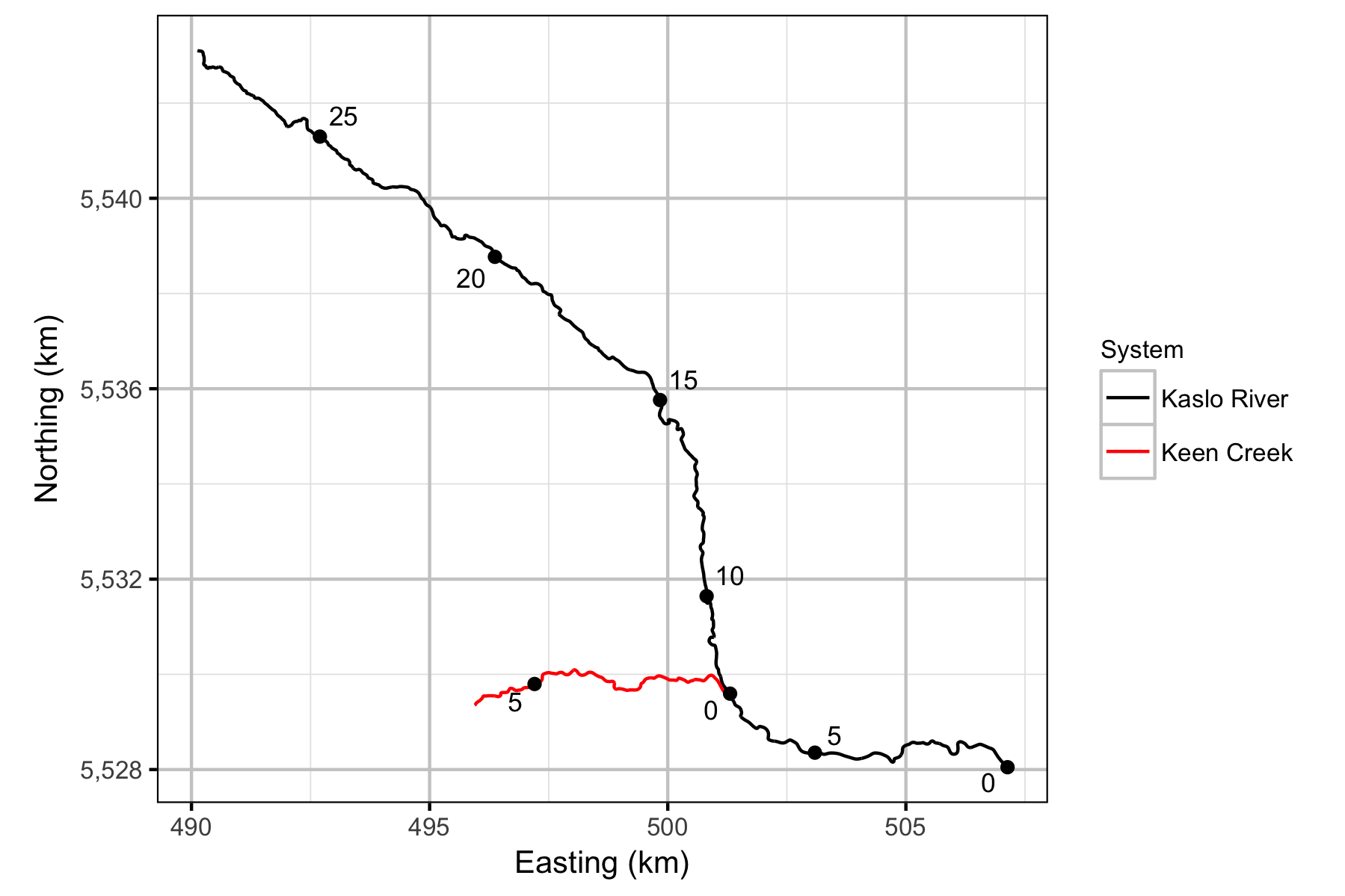Figure 1. Spatial distribution of fish-bearing channel.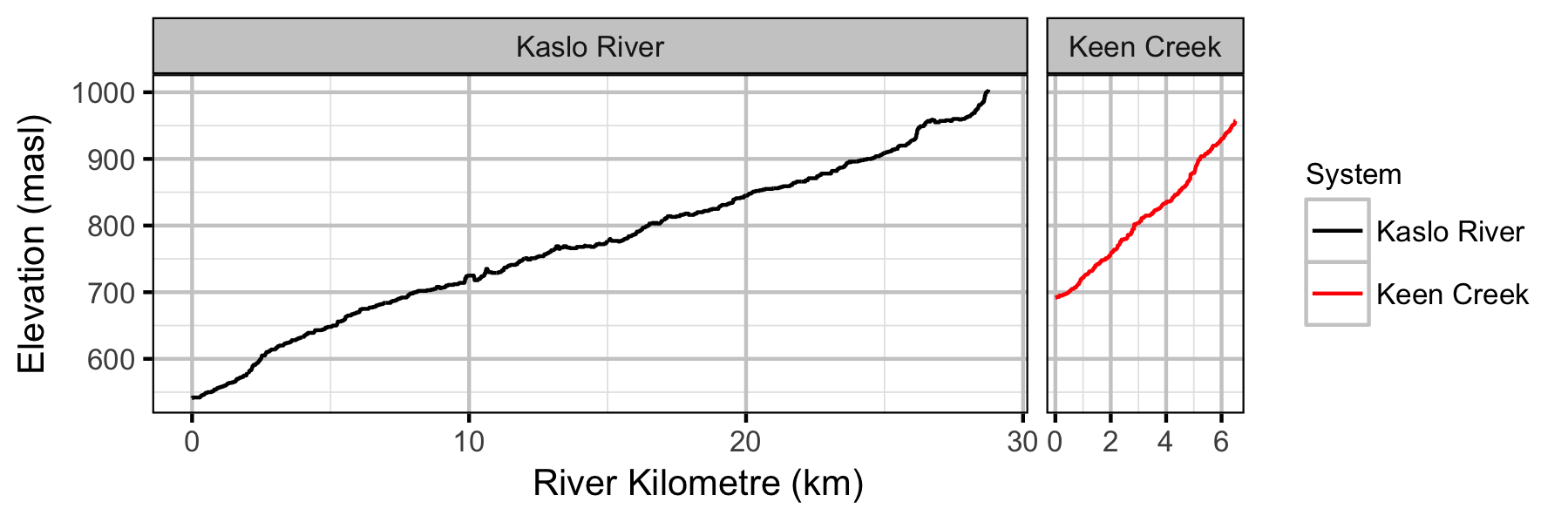Figure 2. Channel elevation by river kilometre and system.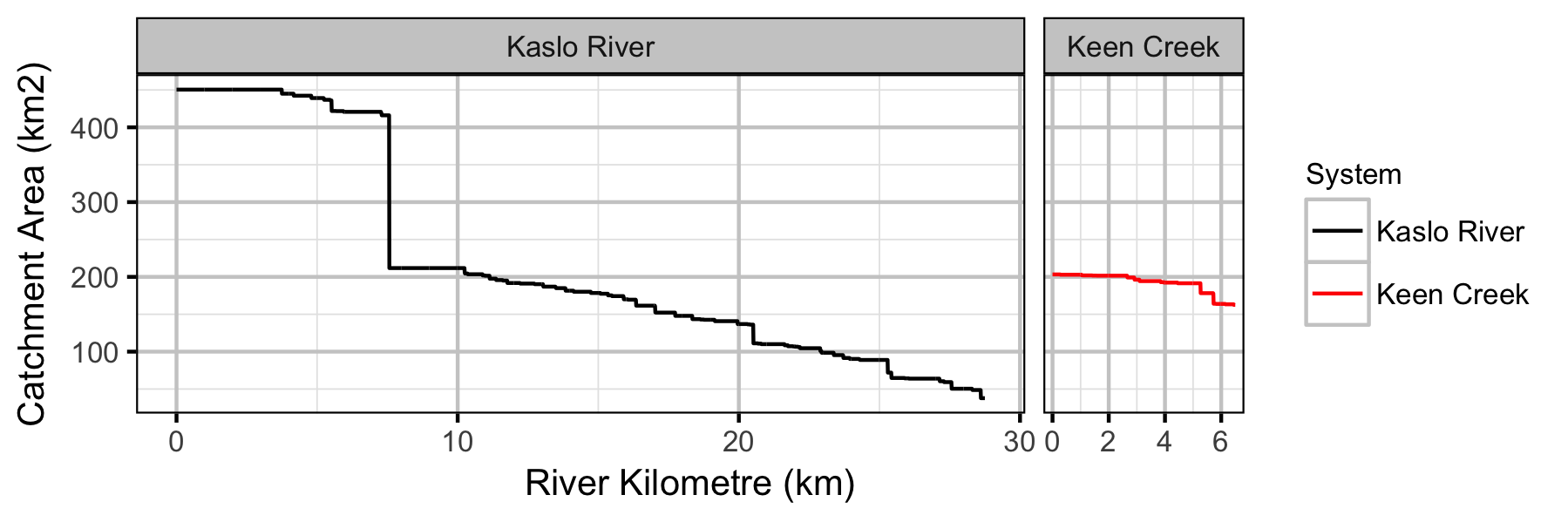Figure 3. Catchment area by river kilometre and system.

### Sites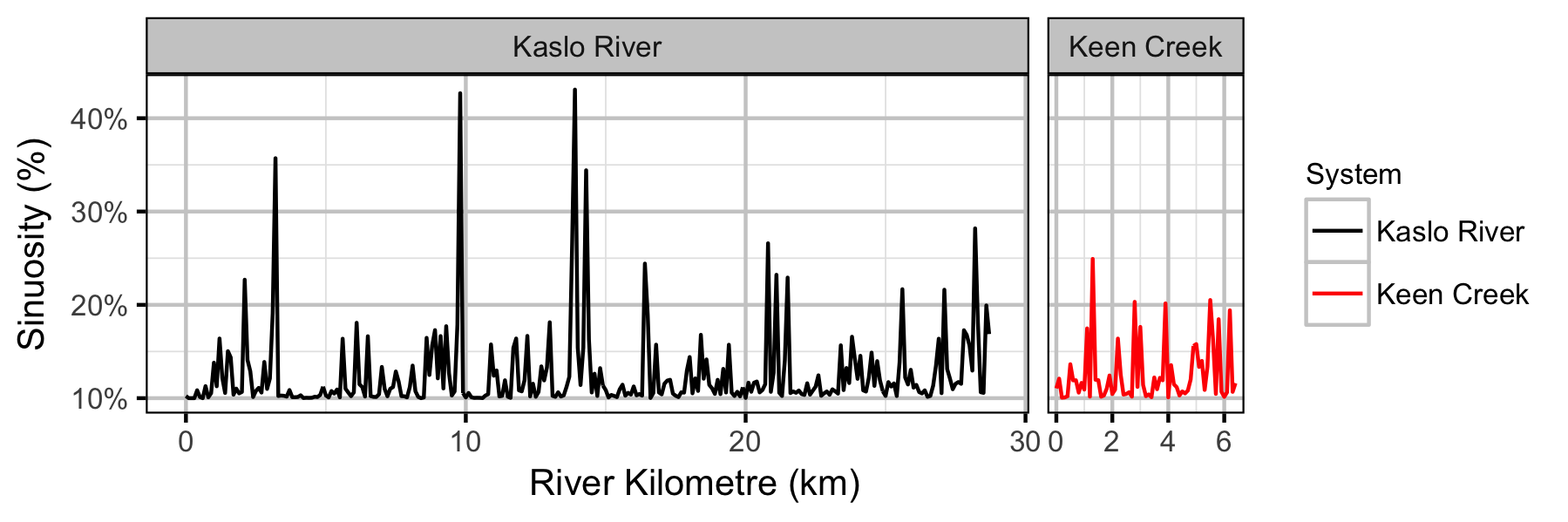Figure 5. Site sinuosity by river kilometre and system.

### Coverage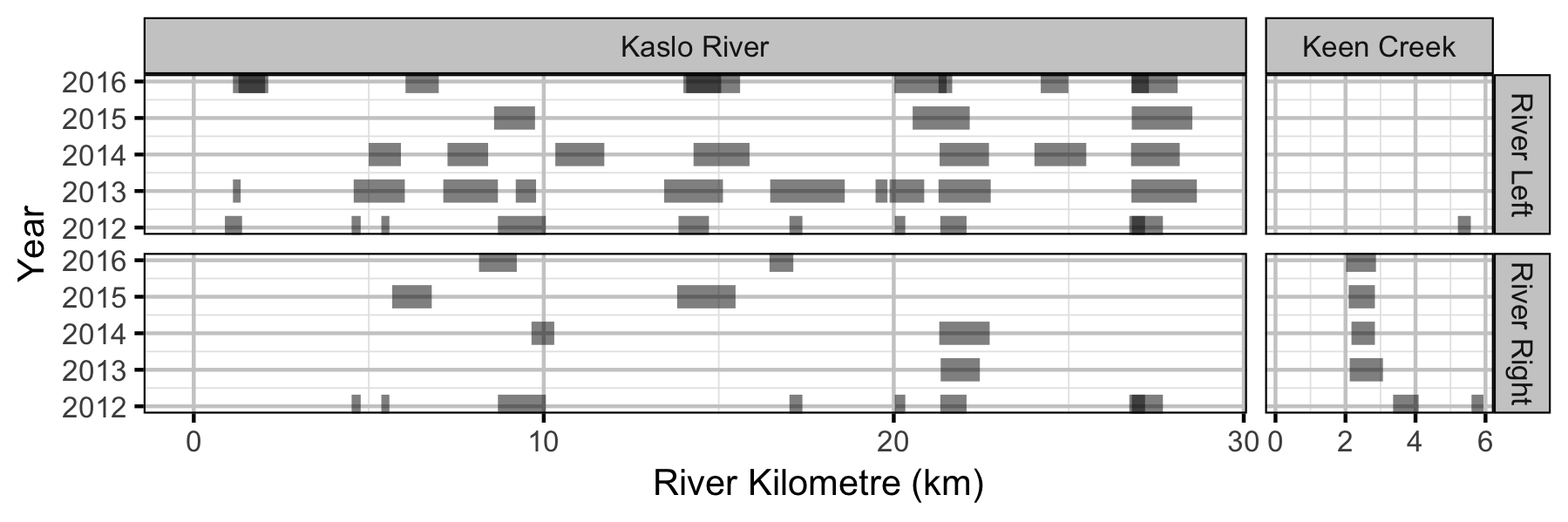Figure 6. Snorkel count coverage by river kilometre, system and bank.

### Fish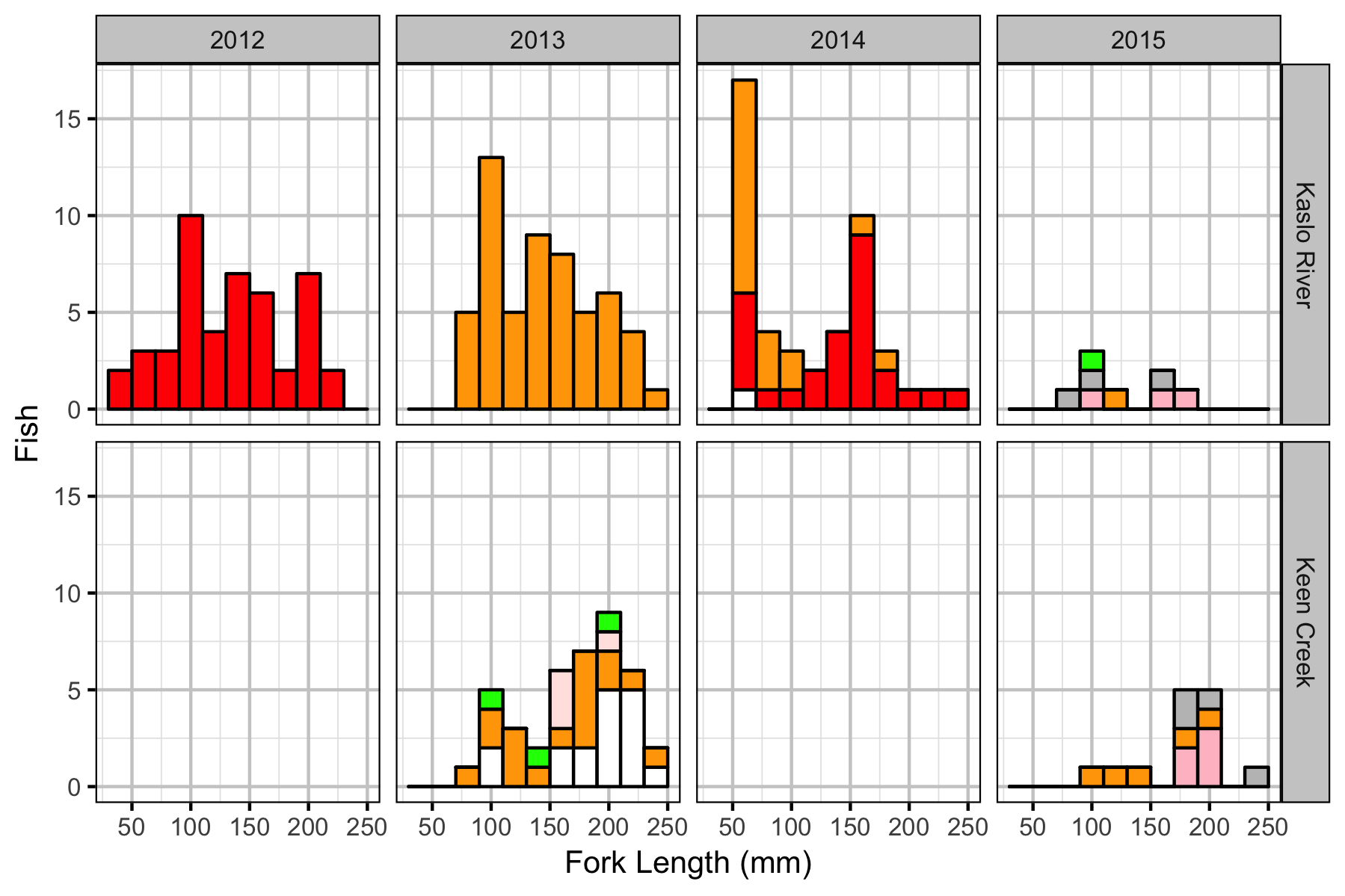Figure 7. Length-frequency plot of marked Bull Trout by year and system.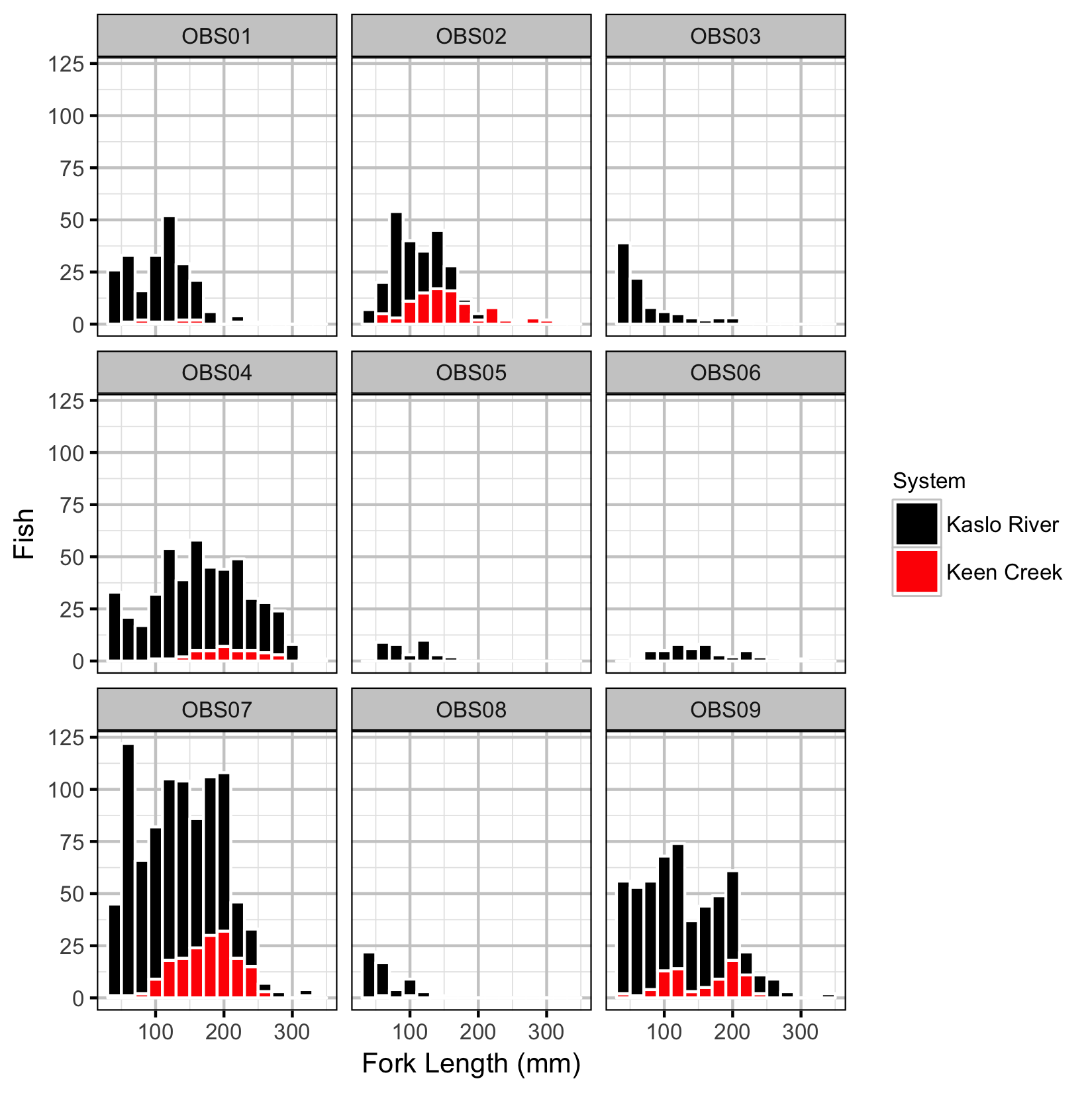Figure 8. Length-frequency plot of counted Bull Trout by observer.

### Observer Efficiency

#### Bayesian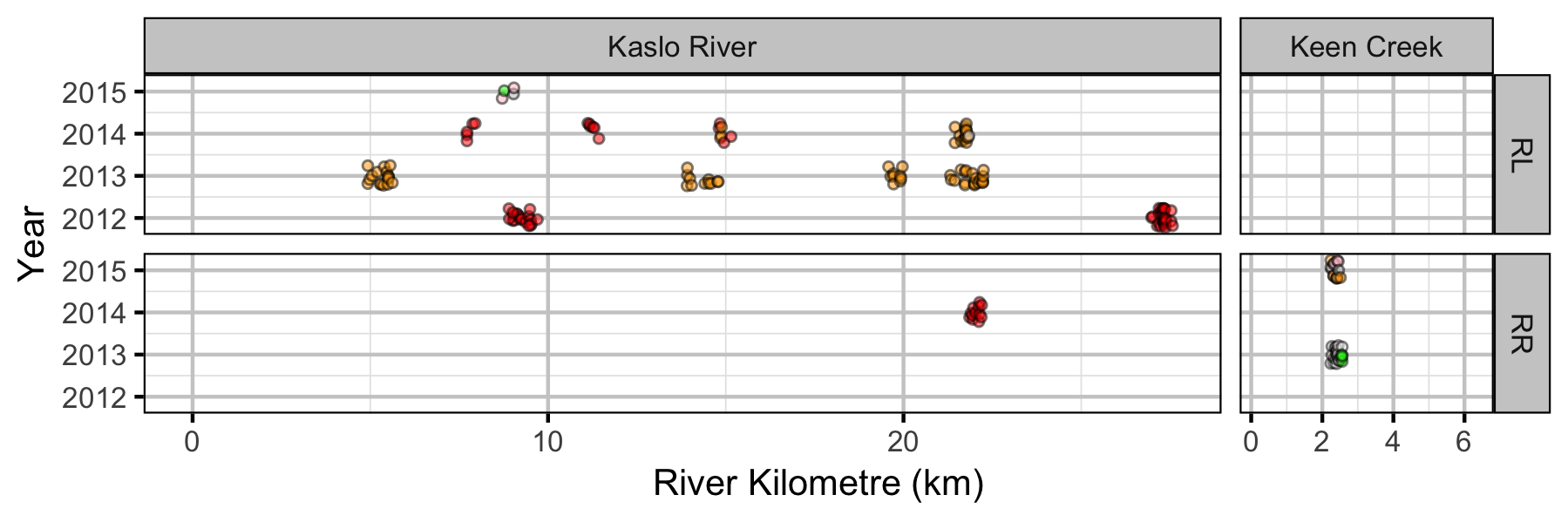Figure 9. Distribution of marked juvenile Bull Trout by year, river kilometre, system and bank.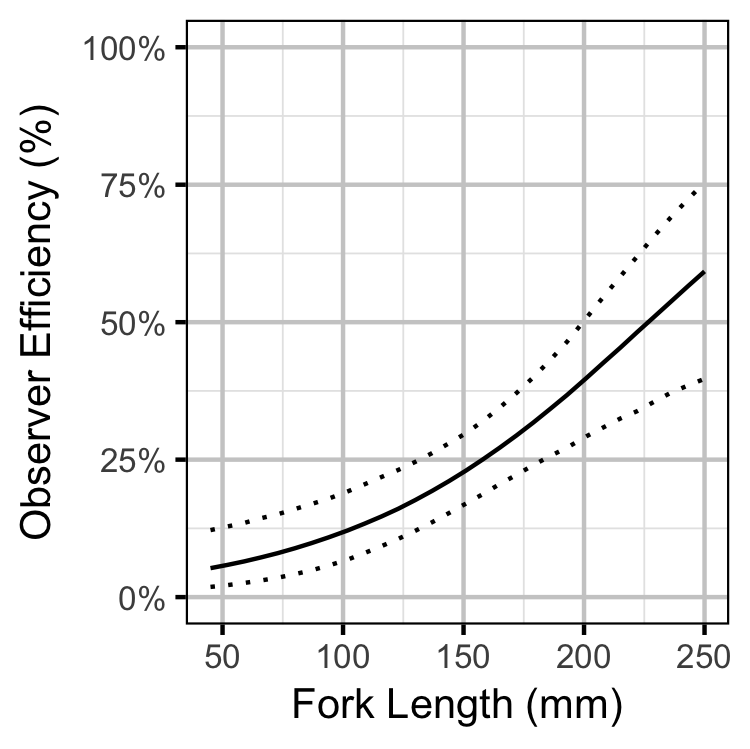Figure 10. Estimated observer efficiency by fork length and system (with 95% CIs).

### Lineal Density

#### Bayesian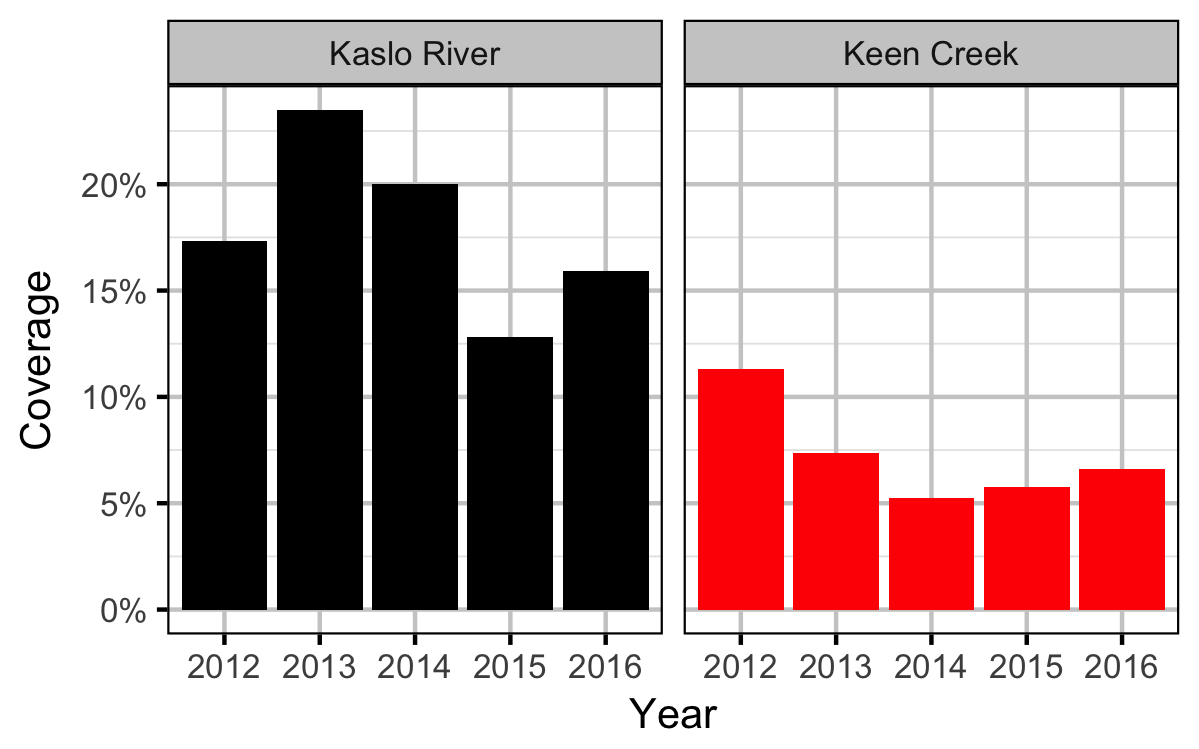Figure 11. Percent coverage by year and system.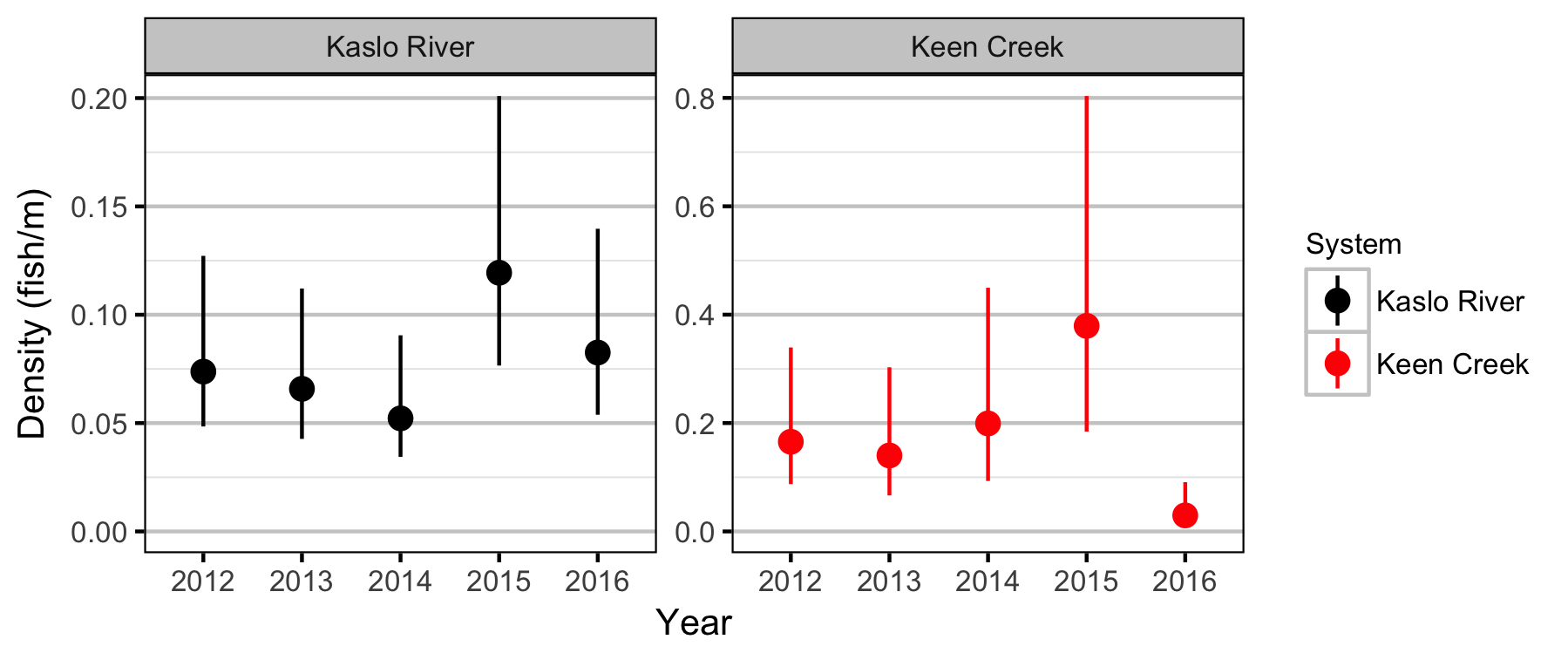Figure 12. Estimated density by year and system (with 95% CIs).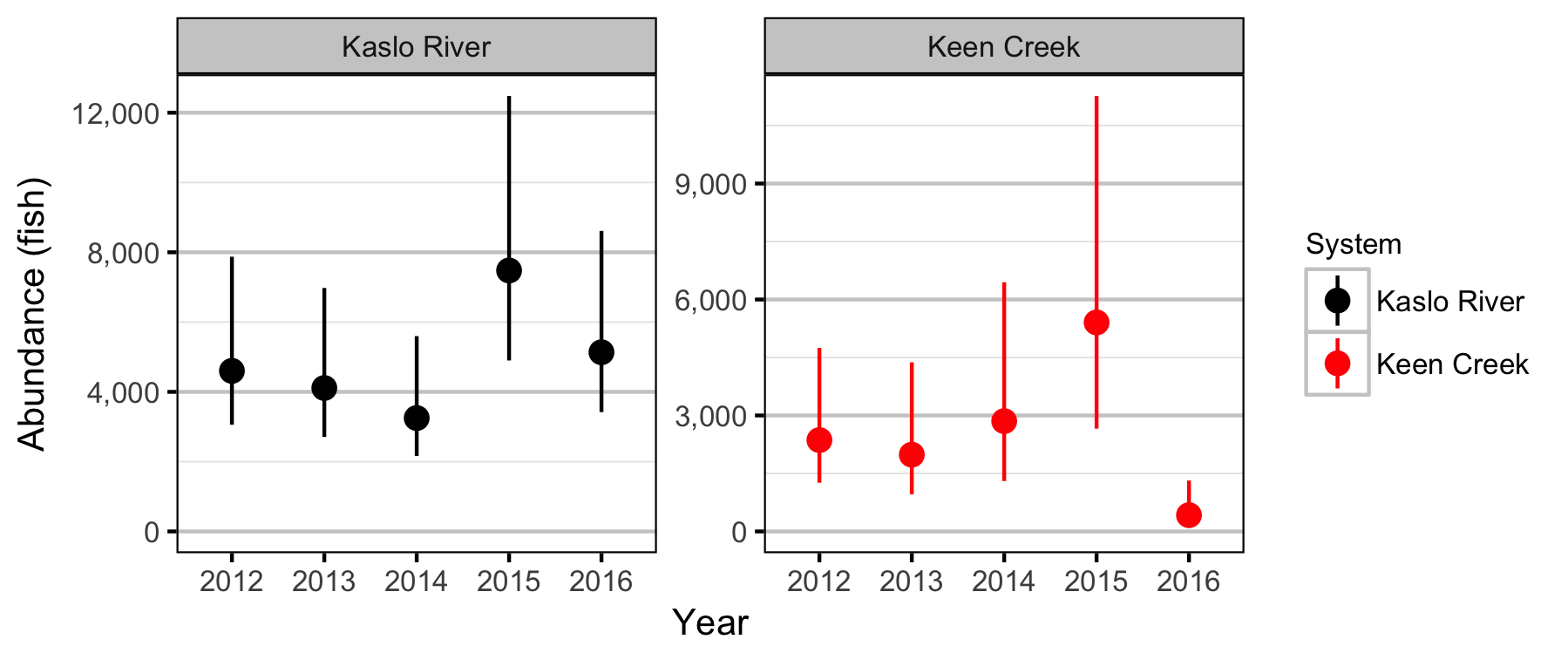Figure 13. The estimated abundance by year and system (with 95% CIs).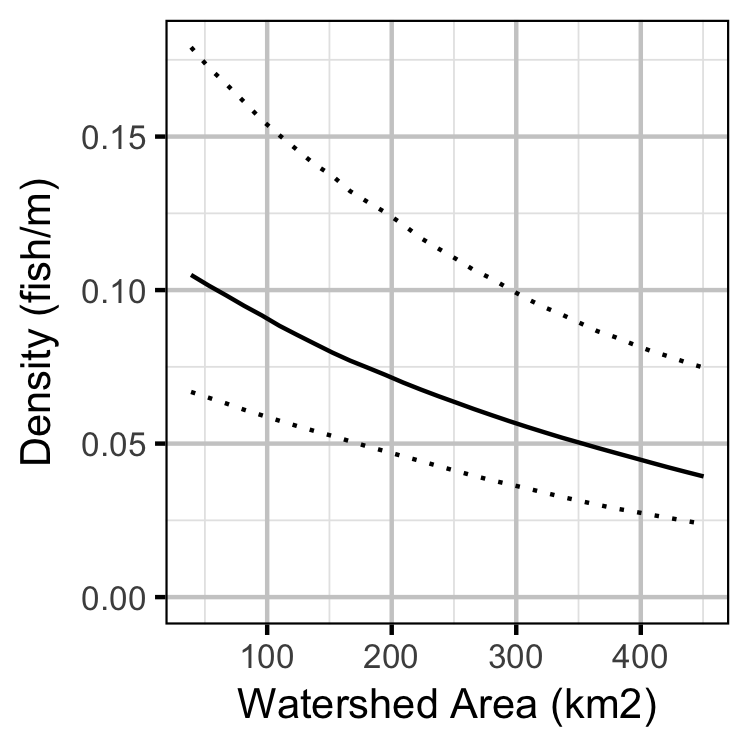Figure 14. The estimated density by watershed area (with 95% CIs).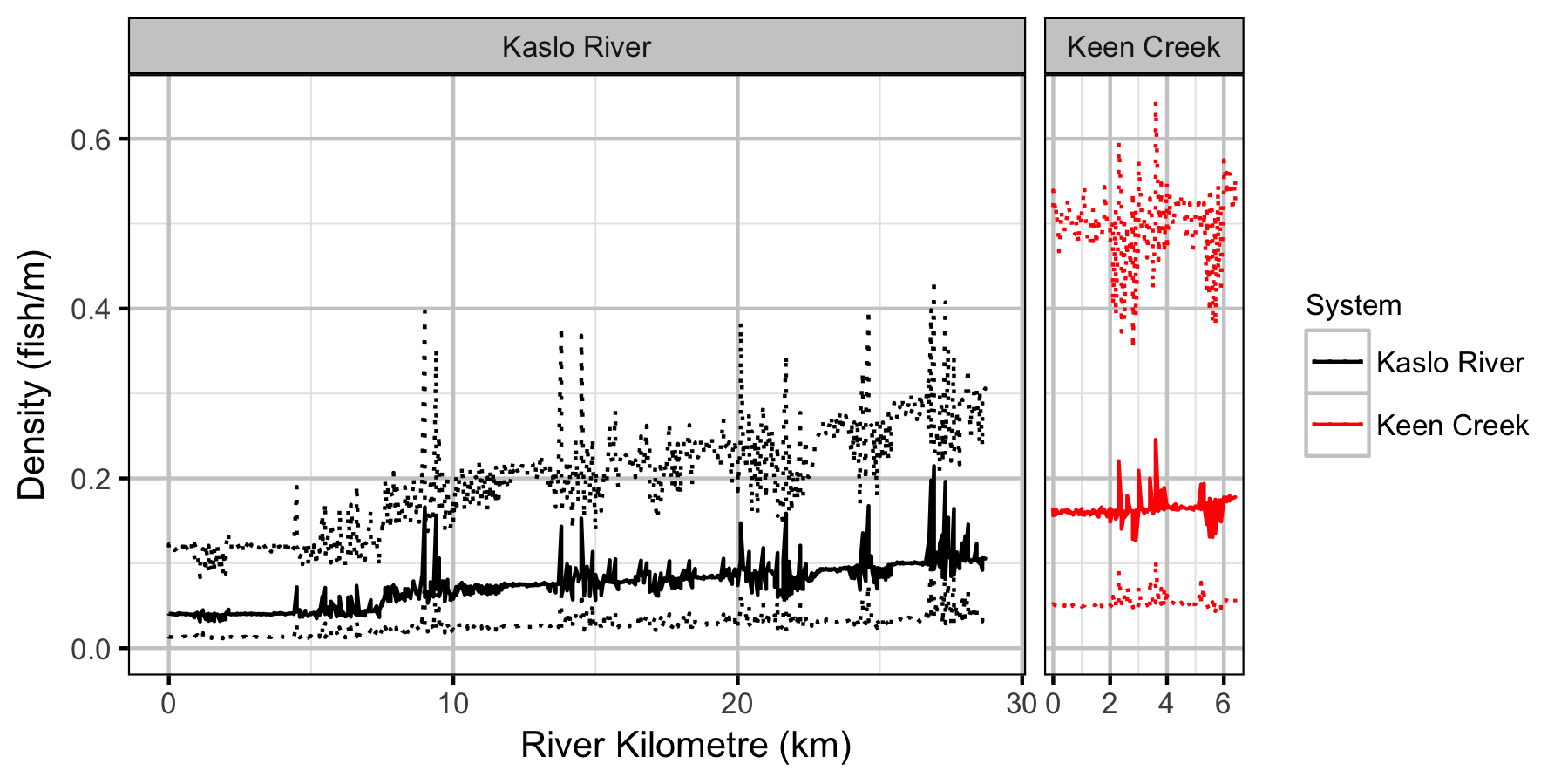Figure 15. The estimated density by site and system (with 95% CIs).

### Stock-Recruiment

#### Bayesian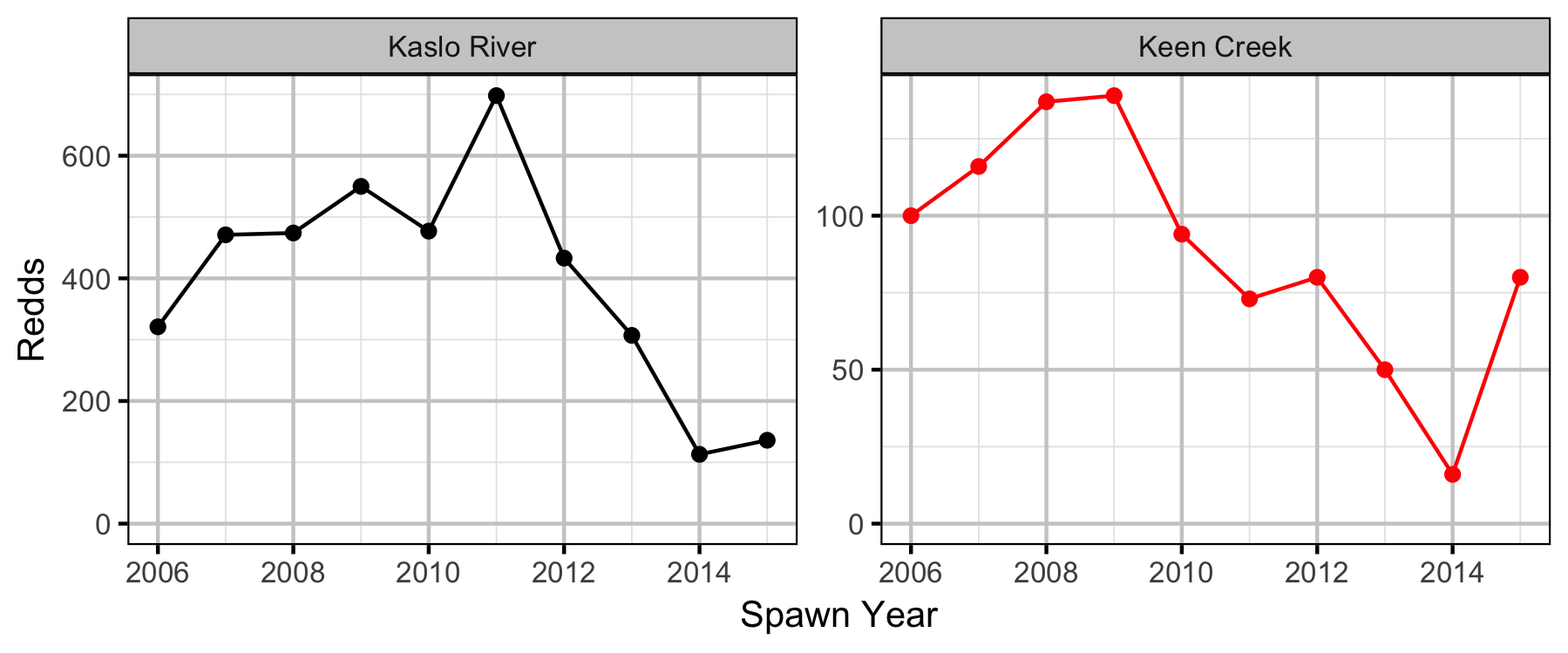Figure 16. Complete redds by system and spawn year.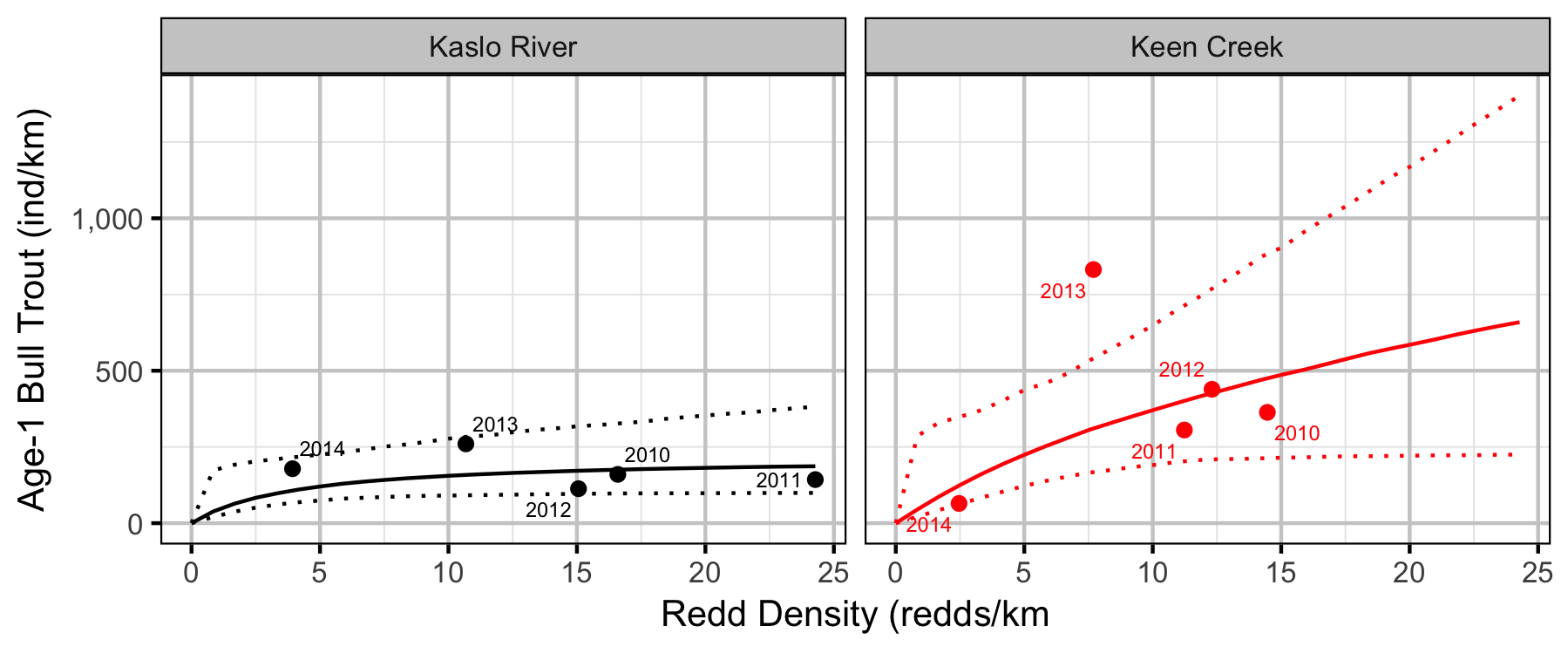Figure 17. Estimated stock-recruitment relationship (with 95% CIs). The points are labelled by spawn year.

## Conclusions

• Observer efficiency is very low for age-0 Bull Trout and increases with size.
• Sinuosity and gradient are not important predictors of the densities of age-1 Bull Trout in Keen Creek and the Kaslo River.

### Recommendations

• Field
• Vary tag colors by fish lengths and locations so it is easy to match resighted fish to individual tagged fish.
• GIS
• Map river locations to the 1 m scale.
• Analytic
• Quantify observer differences in length estimation and efficiency.
• Model autocorrelation in site densities.
• Compare explanatory power of river kilometre versus watershed area.

## Acknowledgements

The organisations and individuals whose contributions have made this analysis report possible include:

• BC Fish and Wildlife
• Greg Andrusak
• Gary Pavan
• Stephan Himmer
• Jimmy Robbins
• Gillian Sanders
• Jason Bowers
• Jeff Berdusco
• Kyle Mace